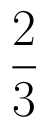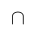Select Page

# CBSE MCQ Maths 12 Science Matrices Answers in English

CBSE MCQ Maths 12 Science Matrices Answers in English to enable students to get Answers in a narrative video format for the specific question.

Expert Teacher provides CBSE MCQ Maths 12 Science Matrices Answers through Video Answers in English language. This video solution will be useful for students to understand how to write an answer in exam in order to score more marks. This teacher uses a narrative style for a question from Matrices not only to explain the proper method of answering question, but deriving right answer too.

Please find the question below and view the Answer in a narrative video format.

Question:

## Similar Questions from CBSE, 12th Science, Maths, Matrices

Question 1 : Find the value of Y, ifand(View Answer Video)

Question 2 : Find the value of x from the equation:. (View Answer Video)

Question 3 :  Find the value of y, if(View Answer Video)

Question 4 : Compute:. (View Answer Video)

Question 5 : LetFind 3A - C. (View Answer Video)

### Inverse Trigonometric Functions

Question 1 : Write the principal value of(View Answer Video)

Question 2 : Write in the simplest form:(View Answer Video)

Question 3 :

Write the value of the following:Question 4 : Write in the simplest form:(View Answer Video)

Question 5 : Write the value of(View Answer Video)

### Probability

Question 1 : If E and F are two events such that,, Find P(not E and not F). (View Answer Video)

Question 2 : If P(F) = 0.35 and=0.85 and E and F are independent events. Find P(E).  (View Answer Video)

Question 3 : A problem in mathematics is given to 4 students A, B, C, D. Their chances of solving the problem, respectively, areand. What is the probability that atmost one of them solve the problem?  (View Answer Video)

Question 4 : A speaks truth in 60% of the cases, while B in 90% of cases. In what percent of cases are they likely to contradict each other in stating the same fact? In the cases of contradiction do you think, the statement B will carry more weight as he speaks truth in more number of cases than A?    (View Answer Video)

Question 5 : Compute P(A/B) if P(B) =0.5 and P(AB)=0.32. (View Answer Video)

### Three Dimensional Geometry

Question 1 : Write the sum of intercepts cut off by the planeon the three axes.  (View Answer Video)

Question 2 : Find the equation of the plane passing through the line of intersection of the planesandwhich is perpendicular to the plane. (View Answer Video)

Question 3 : Find the distance of the point (-1, -5, -10) from the point of intersection of the lineand plane(View Answer Video)

Question 4 : Find the distance of the point (-1, -5, -10) from the point of intersection of the line joining the points A(2, -1, 2) and B(5, 3, 4) with the plane x - y + z = 5. (View Answer Video)

Question 5 : Find the co-ordinates of the point where the line through the points A (3, 4, 1) and B (5, 1, 6) crosses the XY-plane. (View Answer Video)